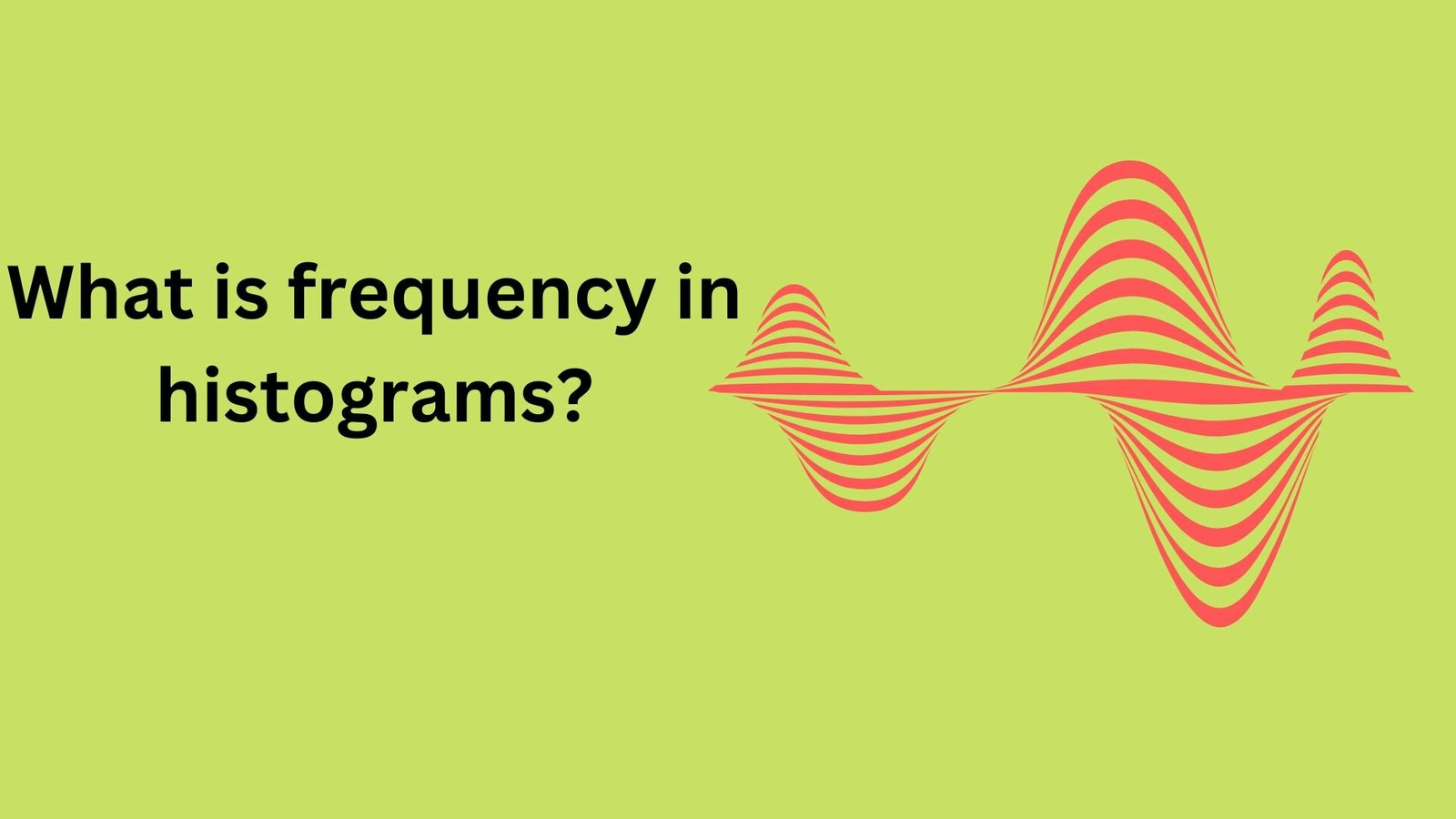# What is frequency in histograms?A histogram is a graph that shows how a set of data is distributed. In a histogram, the frequency of a data point refers to the number of times that data point appears in the set of data. It is represented in the histogram as the height of a bar, with the width of the bar representing the range of values included in that data point.

To create a histogram, the data must first be separated into intervals or bins. These bins reflect the range of values contained in each data point. For example, if we had data on the heights of 100 persons, we could divide the data into 5 centimetre bins, so that one bin represents heights between 145 and 150 cm, another bin represents heights between 150 and 155 cm, and so on. The frequency of a data point is then determined as the number of data values that fall within the bin's range. This frequency is then used to calculate the height of the bar in the histogram that represents that data point.

The histogram is a visual depiction of the distribution of data that allows us to discover patterns and trends that may not be immediately obvious when looking at raw data. For example, if the histogram shows that the majority of the data values are concentrated around a specific range, we can conclude that the data is skewed or skewed to one side. If the histogram is symmetrical and the bars are evenly spaced, we can conclude that the data is roughly regularly distributed.

Histograms can be used to assess the likelihood of particular events in addition to depicting the distribution of data. For example, if we know that the majority of the heights in our data set are between 145 and 155 cm, we may estimate the likelihood that a person chosen at random will be in that range.